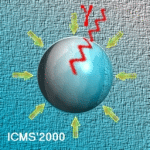# ICMS'2000

The International Conference on Multiphase Systems, ICMS'2000
Ufa, RUSSIA, June 15-17, 2000

Aerosols Particles Formation in Aircraft Jet Plumes

Starik A. M., Savel'ev A. M., Titova N. S.

Central Institute of Aviation Motors, Moscow, RUSSIA

This work presents results of modeling studies of aerosol precursors in the internal flow of gasturbine engine and influence of these precursors ($SO_2$, $SO_3$, $HSO_3$, $H_2SO_4$, $H_2O$) on the sulfate $H_2O/H_2SO_4$ volatile aerosol generation in the near field plume. The mechanisms of chemi-ions formation in combustor and effect of these ions abundance in the nozzle exhaust on positive and negative ion-clusters production in the plume were analyzed too. The evolution of size aerosol distribution and chemical mixture composition change was simulated using developed quasi-one dimensional flow field model with coupled gas phase kinetics, aerosol nucleation, condensation and coagulation processes. It was found that abundance of aerosol precursors $SO_3$, $HSO_3$, $H_2SO_4$ at the nozzle exit leads to higher aerosol number densities, larger total aerosol surface area and increases of a number of larger volatile aerosol size particles in near field plume. Generation of $HSO_4^-$, $NO_3^-$, $NO^+$, $C_2H_3O^+$ ions in combustor results in formation of charged clusters mostly $HSO_4(H_2SO_4)_n$, $NO_3^-(HNO_3)(H_2O)$, $HSO_4^-(HNO_3)_n$, $NO^+(H_2O)_m$, $C_2H_3O_2+(H2O)_m$. Concentration of ion-clusters may reach around 3$\cdot$10$^5$ - 3$\cdot$10$^$6 particles per cm$^3$.